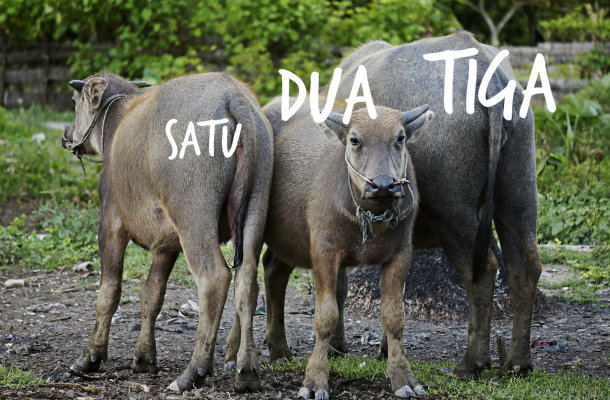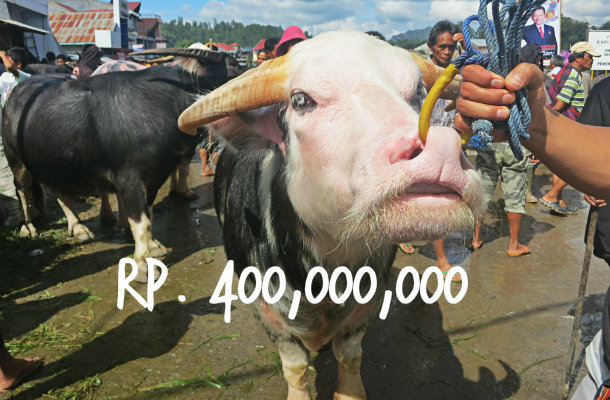Hi there! I would like to continue to the lessons 😀 Oh I already feel like a teacher! Anyways, I was supposed to explain this in the first post about Indonesian Language, but I totally forgot. Bahasa Indonesia, uses the Roman alphabet, from A to Z. The pronunciation of Bahasa is different from English, like I said before it more like Spanish.Before numbers and money, I think a quick look at the pronounciation will be very useful. Lets look at the vowels: a, e, i, o, u
A = Pronounced like “a” in Gelato
E = Pronounced like the “e” in Red
I = Pronounced like the “ee” in Deep
O = Pronounced like the “o” in Stop
U = Pronounced like the “oo” in Moon

### Okay, now I will share Numbers and Money. Are you ready? :p

0 = Zero = Nol
1 = One = Satu
2 = Two = Dua
3 = Three = Tiga
4 = Four = Empat
5 = Five = Lima
6 = Six = Enam
7 = Seven = Tujuh
8 = Eight = Delapan
9 = Nine = Sembilan
10 = Ten = Sepuluh
100 = a hundred = Seratus
1,000 = a thousand = Seribu
100,000 = a hundred thousand = Seratus ribu
200,000 = two hundred thousand = Dua ratus ribu
1,000,000 = a million = Satu juta
2,000,000 = two million = Dua jutaIf we are talking about Indonesian money (IDR = Indonesia Rupiah or we usually said Rupiah = Rp.), many foreigner get really confused, because the Rupiah has so many zeros! Congratulations, you are a millionaire here 🙂 Anyways, to make it easy, some people just remember the colour of the money. For example Rp. 100,000 (Seratus ribu rupiah) = is a red note, Rp. 50,000 (Lima puluh ribu rupiah) = is a blue note. A foreign friend of mine always asks “How many blues / reds do I need to pay for this stuff?”Tip: Rp10,000 is almost US \$1 dollar, so just get rid of four zeros and you will know how much money you are holding. Alternatively one blue note is equal to \$5 and one red is \$10.